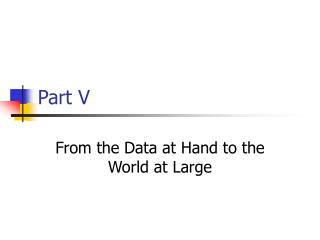Download PresentationPart V

# Part V

Download Presentation## Part V

- - - - - - - - - - - - - - - - - - - - - - - - - - - E N D - - - - - - - - - - - - - - - - - - - - - - - - - - -
##### Presentation Transcript

1. Part V From the Data at Hand to the World at Large

2. Chapter 18Sampling Distribution Models • Modeling the Distribution of sample proportions • From 1000 randomly selected voters (2004) • Poll 1 : John Kerry 49% • Poll 2 : John Kerry 45.9%

3. Assumptions and Conditions • Assumptions • The sampled values must be independent of each other • The sample size, n, must be large. • Conditions • 10% Condition • If drawing without replacement then the sample n must be no larger than 10% of the population • Success / Failure condition • The sample size has to be big enough so that both np and nq are greater than 10

4. The Sampling Distribution Model for a Proportion • Provided that the sampled values are independent and the sample size is large enough, the sampling distribution of p is modeled by a normal model with mean • And standard deviation

5. Models for proportions • Exercise 10 page 424

6. Means: The Fundamental Theorem of Statistics • Central Limit Theorem (CLT) • The sampling distribution of any mean becomes normal as the sample grows (independent observations) • As the sample size “n” increases, the mean of n independent values has a sampling distribution that tends toward a normal model with mean equal to the population mean and standard deviation

7. CLT

8. Assumptions and Conditions • Random Sampling Condition • The values must be sampled randomly • Independence assumption • 10% condition • The sample size is less than 10% of the population

9. Exercise • Step-by-step page 418 • Ex.36 page 426

10. Standard Error • When we estimate the standard deviation of a sampling distribution using statistics found from the data, the estimates are called standard error: • For a proportion • For the sample mean

11. Don’t confuse the sampling distribution with the distribution of the sample • Distribution of the sample • Take a sample • Look at the distribution on a histogram • Calculate summary statistics • Sampling Distribution • Models an imaginary collection of the values that a statistic, might have taken from all the samples that you didn’t get. • We use the sampling distribution model to make statements about how statistics varies

12. Confidence Intervals for Proportions • Example: Infected Sea fan corals at Las Redes Reef (LRR)

13. Confidence Intervals • 68% of the samples will have p^ within 1 SE of p. And 95% of all samples will be within p±2SE • We know that for 95% of random samples p^ will be no more than 2SE away from p. • Now from p^ point of view, there is a 95% chance that p is no more than 2SE away from p^

14. Confidence interval • We are 95% confident that between 42.1% and 61.7% of LRR sea fans are infected. • Margin of Error • Certainty vs. Precision • Estimate ± M.E. • The margin of error for our 95% confidence interval was 2SE • For 99.7% confident 3SE • 100% Confident 0% to 100% • Low Confidence 51.8% to 52.0

15. Critical Values z* • The number of standard errors to move away from the mean of the sampling distribution to correspond to the specified level of confidence. • Find z* (critical value) for 98% confidence. • For 95%?

16. Confidence interval(one-proportion z-interval) • The critical value z* depends on the particular confidence interval we specify and • Assumptions • Independence • Conditions • Randomization • 10% Condition

17. Exercise • #13 Page 444

18. Chapter 20 Testing Hypothesis about proportions • Example: • Metal Manufacturer • Ingots • 20% defective (cracks) • After Changes in the casting process: • 400 ingots and only 17% defective • IS this a result of natural sampling variability or there is a reduction in the cracking rate?

19. Hypotheses • We begin by assuming that a hypothesis is true (as a jury trial). • Data consistent with the hypothesis: • Retain Hypothesis • Data inconsistent with the hypothesis: • We ask whether they are unlikely beyond reasonable doubt. • If the results seem consistent with what we would expect from natural sampling variability we will retain the hypothesis. But if the probability of seeing results like our data is really low, we reject the hypothesis.

20. Testing Hypotheses • Null Hypothesis H0 • Specifies a population model parameter of interest and proposes a value for this parameter • Usually: • No change from traditional value • No effect • No difference • In our example H0:p=0.20 • How likely is it to get 0.17 from sample variation?